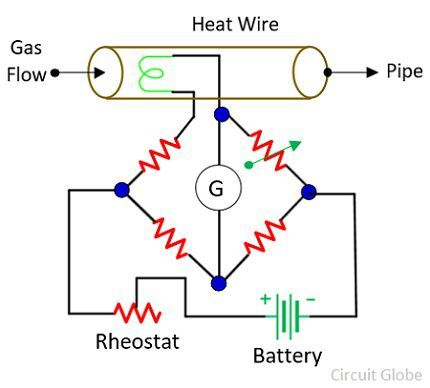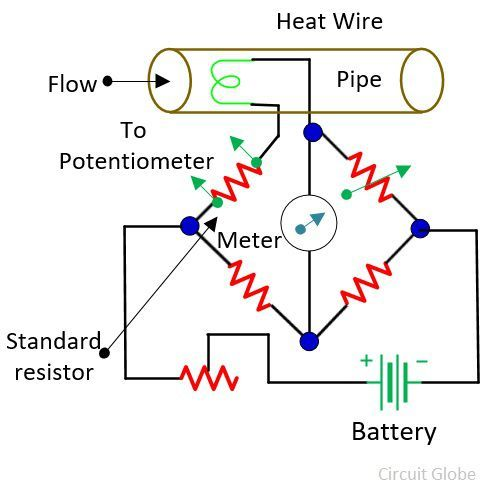# Hot Wire Anemometer

Definition: The Hot Wire Anemometer is a device used for measuring the velocity and direction of the fluid. This can be done by measuring the heat loss of the wire which is placed in the fluid stream. The wire is heated by electrical current.

The hot wire when placed in the stream of the fluid, in that case, the heat is transferred from wire to fluid, and hence the temperature of wire reduces. The resistance of wire measures the flow rate of the fluid.

The hot wire anemometer is used as a research tool in fluid mechanics. It works on the principle of transfer of heat from high temperature to low temperature.

## Construction of Hot Wire Anemometer

The hot wire anemometer consists two main parts.

1. Conducting wire
2. Wheat stone bridge.

The conducting wire is housed inside the ceramic body. The wires are taking out from the ceramic body and connecting to the Wheatstone bridge. The wheat stone bridge measures the variation of resistance.

### Constant Current Method

In the constant current method, the anemometer is placed in the stream of the fluid whose flow rate needs to be measured. The current of constant magnitude is passed through the wire. The Wheatstone bridge is also kept on the constant voltage.When the wire is kept in the stream of liquid, in that case, the heat is transferred from the wire to the fluid. The heat is directly proportional to the resistance of the wire. If heat reduces, that means the resistance of wire also reduces. The Wheatstone bridge measures the variation in resistance which is equal to the flow rate of the liquid.

### Constant Temperature Method

In this arrangement, the wire is heated by the electric current. The hot wire when placed in the fluid stream, the heat transfer from wire to the fluid. Thus, the temperature of the wire changes which also changes their resistance. It works on the principle that the temperature of the wire remains constant. The total current requires to bring the wire in the initial condition is equal to the flow rate of the gas.### Measurement of the rate of a fluid using a Hot Wire Instrument

In hot wire anemometer, the heat transferred electrically to the wire which is placed in the fluid stream. The Wheatstone bridge is used for measuring the temperature of wire regarding their resistance. The temperature of the wire remains constant for measuring the heating current. Thus, the bridge remains balanced.The standard resistor is connected in series with the heating wire. The current across the wire is determined by knowing the voltage drop across the resistor. And the value of voltage drop is determined by the potentiometer.

The equation determines the heat loss from the heated wireWhere, v – velocity of heat flow,
ρ – the density of fluid,

The a and b are the constants. Their value depends on the dimension and the physical properties of the fluid and wire.

Suppose I, is the current of the wire and the R is their resistance. In equilibrium condition,

Heat generated = Heat LostThe resistance and temperature of the instrument are kept constant for measuring the rate of the fluid by measuring the current I.